# Difference between revisions of "Frequency Domain Analysis"

 Prev: Transfer Functions Chapter 9 - Frequency Domain Analysis Next: PID Control

In this chapter we study how how stability and robustness of closed loop systems can be determined by investigating how signals propagate around the feedback loop. The Nyquist stability theorem is a key result that provides a way to analyze stability and introduce measures of degrees of stability.

## Textbook Contents

• 1. Introduction
• 2. The Nyquist Criterion
• 3. Stability Margins
• 4. Bode's Relations
• 5. The Notion of Gain
• 7. Exercises

## Chapter Summary

This chapter describes the use of the Nyquist criterion for determining the stability of a system:

1. The loop transfer function of a feedback system represents the transfer function obtained by breaking the feedback loop and computing the resulting transfer function of the open loop system. For a simple feedback system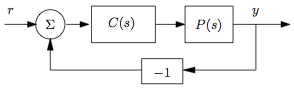the loop transfer function is given by2. The Nyquist criterion provides a way to check the stability of a closed loop system by looking at the properties of the loop transfer function. For a stable open loop system, the Nyquist criterion states that the system is stable if the contour of the loop transfer function plotted fromtohas no net encirclements of the pointwhen it is plotted on the complex plane.

3. The general Nyquist criterion uses the image of the loop transfer function applied to the Nyquist countourThe number of unstable poles of the closed loop system is given by the number of open loop unstable poles plus the number of clockwise encirclements of the point.

4. Stability margins describe the robustness of a system to perturbations in the dynamics. We define the phase crossover frequency,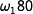as the smallest frequency where the phase of the loop transfer function is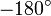and the gain crossover frequency,as the small frequency where the loop transfer function has unit magnitude. The gain margin and phase margin are given byThese margins describe the the maximum variation in gain and phase in the loop transfer function under which the system remains stable. Two other margins are the stability margin, which is the shortest distance frmo the Nyquist curve to the critical point, and the delay margin, which is the smallest time delay required to make the system unstable.

5. Bode's relations relate the gain and phase of a transfer function with no poles or zeros in the right half plane. They show that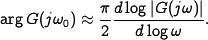A non-minimum phase sytem is one for which there is more phase lag than the amount given by Bode's relations. Systems with right have plane poles or zeros are non-minimum phase.

6. The gain of an input/output system is defined aswhere sup is the supremum. The small gain theorem states that if two systems with gains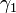andare connected in a feedback loop, then the closed loop system is stable if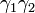.